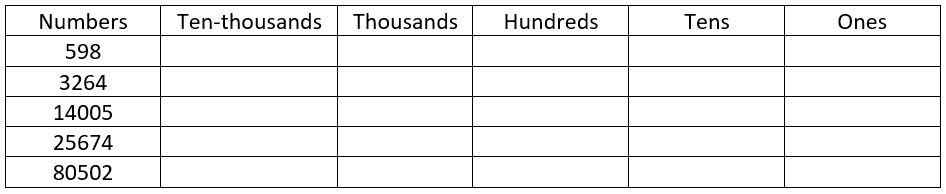## LetsPlayMaths.Com

WELCOME TO THE WORLD OF MATHEMATICS

# Class 3 Number System Worksheet - 5

1. Arrange the digits in the place value table.2. Find the place value

a) 5 in 4579 = __________

b) 9 in 598 = __________

c) 8 in 80597 = __________

d) 3 in 98543 = __________

3. Write the place value of 6 in 35461 = __________

4. Write the place value of 0 in 7098 = __________

5. Write the place value of 4 in 46570 = __________

6. Write True or False

a) The place value of 7 in 7345 is 700. __________

b) The place value of 9 in 90567 is 9000. __________

c) The place value of 5 in 5679 is 5000. __________

d) The place value of 0 in 708 is 0. __________

e) The place value of 6 in 54368 is 60. __________

7. What is the face value of 4 in 94786? __________

8. If we interchange the position of 4 & 8 in 94786, then place value of 4 is _____ and Place value of 8 is _____.

If you want to download the above worksheet, please click below link.

Number System-5Download the pdf

Number System Worksheet - 1

Number System Worksheet - 2

Number System Worksheet - 3

Number System Worksheet - 4

Number System Worksheet - 6

Number System Worksheet - 7

Number System Worksheet - 8

Number System Worksheet - 9

## Answer Sheet

Number-System-AnswerDownload the pdf

Copyright © 2021 LetsPlayMaths.com. All Rights Reserved.